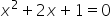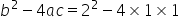Mathematics
Easy

Question

# How many solutions are there for the equationusing the discriminant?

## 0    1    2    Either 1 or 2.Hint:

## The correct answer is: 1

### Step 1 of 1:We have given a quadratic equation= 0The discriminant value is equal to zero, so it has one real solution.

The discriminant is the part of the quadratic formula found within the square root.

### Related Questions to study#### With Turito Foundation.#### Get an Expert Advice From Turito.